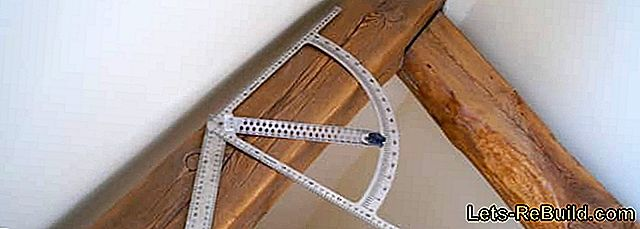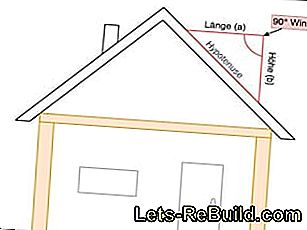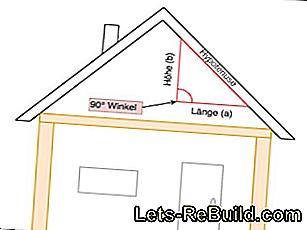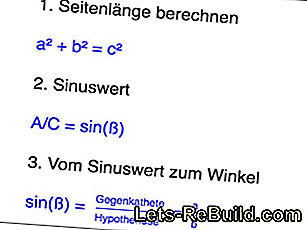# Calculate roof pitch##### Aid for calculating the roof pitch
• Ruler, meter, rangefinder or tape measure
• calculator
• spirit level
• protractor

## Step 1: Dimensions for calculating the roof pitch

The easiest way to measure length (a) and height (b) for the calculation is on an accessible loft. If this is not possible, the dimensions must be taken on the outside of the roof.

But do not worry, for your calculation, you do not have to measure the entire rafter length of the roof structure, for the calculation of the inclination, a section of the slope is already sufficient.

Measure the length (a) and height (b) from any point for a right triangle. Important is the exactCompliance with a 90 degree angle between the length and the height. It is therefore advisable to mark the point from which you are measuring and to use a spirit level or protractor.

Not only in the pointed saddle roof you can determine the dimensions. Even with a pent roof or carport you form a hypothetical triangle with height, length and incline to determine the roof pitch.Or you measure on the outside of the roof.Measure either inside the roof slope.

## Step 2: Formula for calculating the roof pitch

The roof pitch opposite the right angle, ie the longest side of the triangle, forms the hypotenuse. Length (a) and height (b) are called catheters. The right triangle calculate with thePythagorean theorem (see picture). From the result, you then determine thesine value which, in turn, with a unit circle, or more simply, the calculator, in thetilt angle converts. The calculator must be switched to Degree (DEG) to calculate the sine value, and you must work with arcsin.

Do you want theseRoof pitch in percent Express, you can convert the angle degree accordingly. When converting an angle specification, one assumes that one100% incline at a 45° angle of inclination equivalent.

Using the mathematical formula below, you can convert the inclination angle to percent. If you change the formula accordingly, the inclination angle can be calculated in degrees of percentage slope (see picture).Formula for calculating the roof pitch in degrees.Formula for converting degrees to percent and vice versa.

## Step 3: Help to calculate the roof pitchDetermine roof pitch without calculation using an inclinometer app.

The internet offers variousOnline Calculator, which calculate the inclination angle in just one step after entering the required dimensions. Also for the conversion of degrees in percent and vice versa, there are a variety of online tools.

To determine the roof pitch without any measure, you have differentApps for your mobile to disposal. They work on the same principle as professional,digital protractor, The device is simply placed on the slope and displays the tilt angle on the display.

The safest variant, especially for high, inaccessible roofs, is the contracting of a roofer who determines the dimensions for you and precisely calculates the roof pitch.

Author: Yvonne Baldwin

Photo Credits:
• all pictures: Yvonne Bräunig

### Similar sites

• Make shelf yourself
• Tinker lampshade yourself
• Calculate rafters
• Building pallet chairs - construction manual for garden armchairs made of pallets
• Make picture frame yourself
• Instructions: Build your own table in Chabby-Chic
• Shutters yourself - building instructions for shutters
• Build stable and simple wooden staircase yourself
• Make lampshade from hanger yourself
• Building instructions for birdhouses
• Build sideboard shelf - blueprint with construction manual
• Cushion the seat itself
• Build practical wardrobe furniture and wall shelves
• Build your own game tower: Spielhaus construction manual
• Calculate steel weight